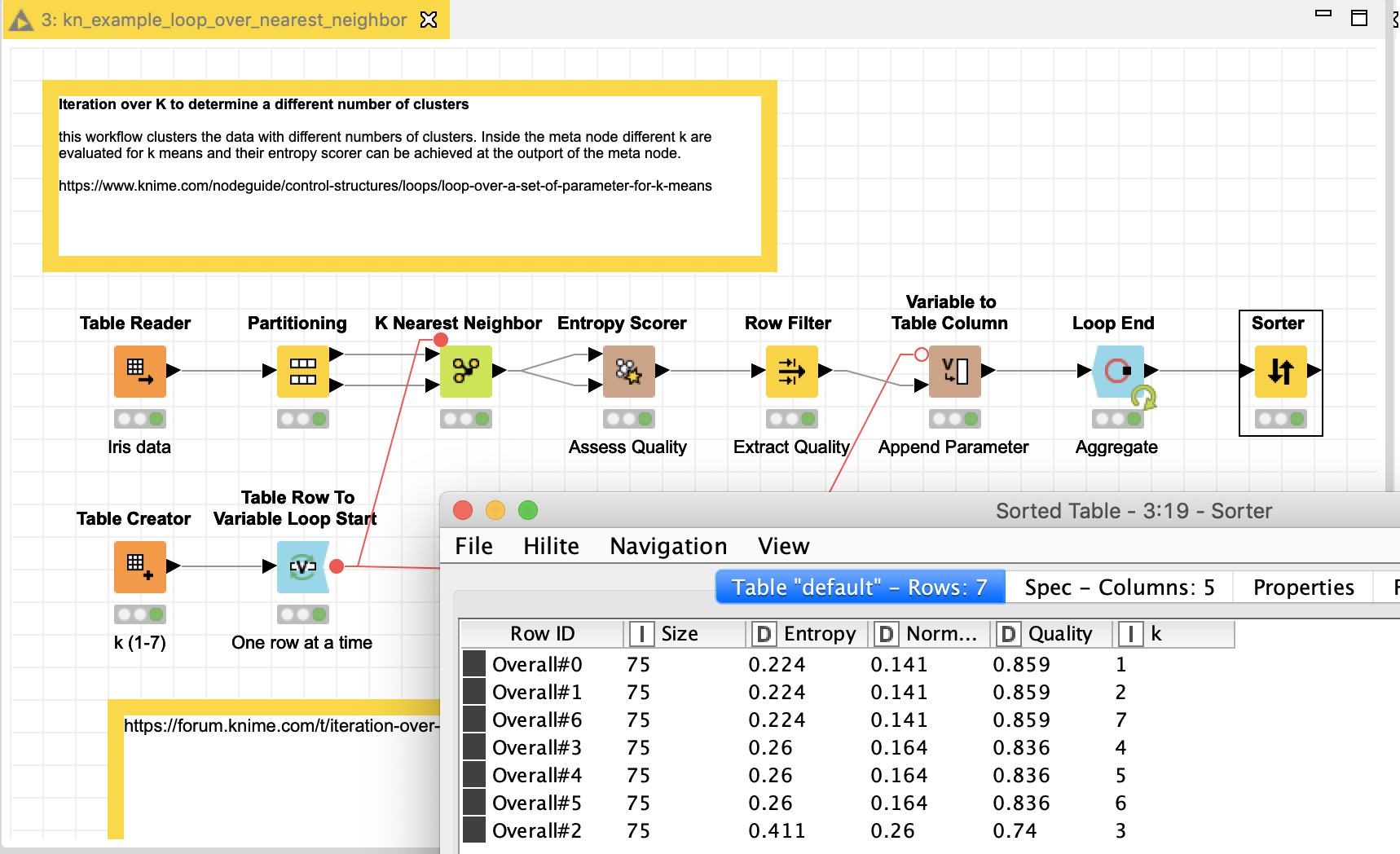# iteration over k values using variables in the K Nearest Neighbor node

Hi - I am trying to control the k value in the K Nearest neighbor from a variable so I can generate a table of various k values and the corresponding accuracy values.

This idea came from the sample 04_Loops/01_Loop_over_a_set_of_parameter_for_k_means. In that sample, the values for k were generated by Table Creator and then fed into Table Row To Variable Loop Start, and the number of clusters in the k-Means node uses that variable.
I use the same approach in the K Nearest Neghbor node, but I get the error: Can’t merge FlowVariables Stacks (likely a loop problem).

Any idea how to parameterize the k values in the K Nearest Neighbor node?

Thank you.

Maybe like this. I have not checked if the results from the Iris sample data would be of any practical use in this scenario.1 Like

Ah yes, thank you - that works. What I was trying to do earlier was to use the n-fold Cross Validation (the X-partitioner) in front of the K Nearest Neighbor node. I guess I can’t do this type of ‘loop within loop’ operationThank you again!